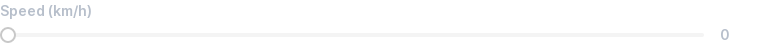# Slider¶

Use sliders to allow picking a number in a given range.

## Basic¶

Set `mode='range'` to show a slider.

The default range is between `0` and `10`.

``````speed = view(box('Speed (km/h)', mode='range'))
``````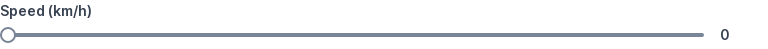## Set initial value¶

Set `value=` to default the slider value.

``````speed = view(box('Speed (km/h)', mode='range', value=5))
``````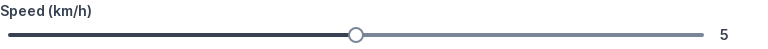## Set min value¶

Set `min=` to specify a minimum value.

``````speed = view(box('Speed (km/h)', mode='range', min=3))
``````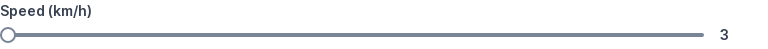## Set max value¶

Set `max=` to specify a maximum value.

``````speed = view(box('Speed (km/h)', mode='range', max=100))
``````## Set step¶

Set `step=` to specify how much to increment or decrement by.

The default step is `1`.

``````speed = view(box('Speed (km/h)', mode='range', step=2))
``````## Set precision¶

Set `precision=` to specify how many decimal places the value should be rounded to.

The default is calculated based on the precision of step:

• if step = 1, precision = 0
• if step = 0.42, precision = 2
• if step = 0.0042, precision = 4
``````speed = view(box('Speed (m/s)', mode='range', value=0.6, min=-2, max=2, step=0.2, precision=2))
``````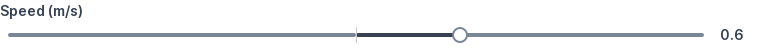## Combine min, max, step, precision¶

`min=`, `max=`, `step=` and `precision=` can be combined.

``````speed = view(box('Speed (km/h)', mode='range', min=10, max=100, step=5))
``````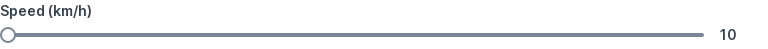## Set range¶

Set `range=` to a `(min, max)` tuple to restrict numeric inputs between two values.

This is a shorthand notation for setting `min=` and `max=` individually.

``````speed = view(box('Speed (km/h)', mode='range', range=(10, 100)))
``````## Set range with step¶

Set `range=` to a `(min, max, step)` tuple to increment/decrement by steps other than `1`.

This is a shorthand notation for setting `min=`, `max=` and `step` individually.

``````speed = view(box('Speed (km/h)', mode='range', range=(10, 100, 5)))
``````## Set range with precision¶

Setting `range=` to a `(min, max, step, precision)` tuple is shorthand setting `min=`, `max=`, `step` and `precision` individually.

``````speed = view(box('Speed (m/s)', mode='range', value=0.6, range=(-2, 2, 0.2, 2)))
``````## Zero-crossing range¶

Ranges can cross zero.

``````speed = view(box('Speed (m/s)', mode='range', value=-3, range=(-5, 5)))
``````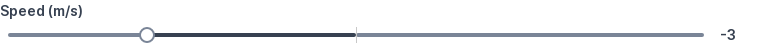## Set fractional steps¶

Steps can be fractional.

``````speed = view(box('Speed (m/s)', mode='range', value=0.6, range=(-2, 2, 0.2)))
``````## Handle changes immediately¶

Add `live` to `mode` to handle changes immediately.

``````speed = 5  # Starting value
while True:
speed = view(
box('Speed (km/h)', mode='live range', value=speed),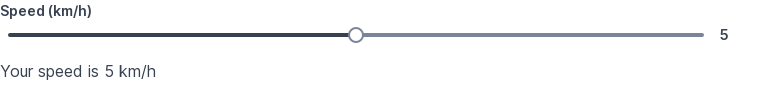Set `disabled=True` to disable.
``````view(box('Speed (km/h)', mode='range', disabled=True))